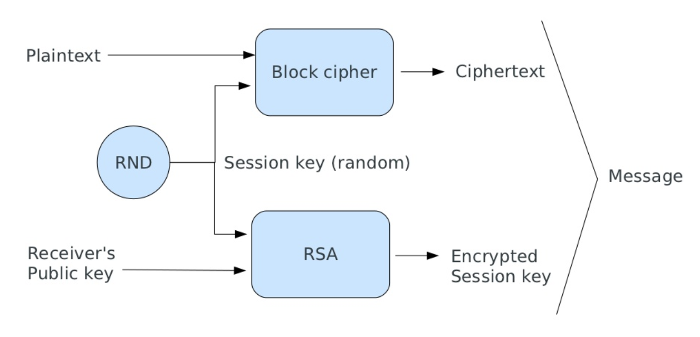# Hybrid Cryptosystem

Available since version 3.1.0

Hybrid is an encryption mode that uses symmetric and public key ciphers together. The approach takes advantage of public key cryptography for sharing keys and symmetric encryption speed for encrypting messages.

Hybrid mode allows you to encrypt a message for one or more receivers, and can be used in multi-user scenarios where you wish to limit decryption to specific users.

## How it works

Suppose we have two users: Alice and Bob. Alice wants to send a message to Bob using a hybrid cryptosystem, she needs to:

• Obtain Bob's public key;
• Generates a random session key (one-time pad);
• Encrypts message using a symmetric cipher with the previous session key;
• Encrypts session key using the Bob's public key;
• Sends both the encrypted message and encrypted session key to Bob.

A schema of the encryption is reported in the image below:To decrypt the message, Bob needs to:

• Uses his private key to decrypt the session key;
• Uses this session key to decrypt the message.

## Example of Usage

In order to use the `Zend\Crypt\Hybrid` component, you need to have a keyring of public and private keys. To encrypt a message, use the following code:

``````use Zend\Crypt\Hybrid;
use Zend\Crypt\PublicKey\RsaOptions;

// Generate public and private key
\$rsaOptions = new RsaOptions([
'pass_phrase' => 'test'
]);
\$rsaOptions->generateKeys([
'private_key_bits' => 4096
]);
\$publicKey  = \$rsaOptions->getPublicKey();
\$privateKey = \$rsaOptions->getPrivateKey();

\$hybrid     = new Hybrid();
\$ciphertext = \$hybrid->encrypt('message', \$publicKey);
\$plaintext  = \$hybrid->decrypt(\$ciphertext, \$privateKey);

printf(\$plaintext === 'message' ? "Success\n" : "Error\n");
``````

We generated the keys using the Zend\Crypt\PublicKey\RsaOptions component. You can also use a PEM string for the keys. If you use a string for the private key, you need to pass the pass phrase to use when decrypting, if present, like in the following example:

``````use Zend\Crypt\Hybrid;
use Zend\Crypt\PublicKey\RsaOptions;

// Generate public and private key
\$rsaOptions = new RsaOptions([
'pass_phrase' => 'test'
]);
\$rsaOptions->generateKeys([
'private_key_bits' => 4096
]);
// Strings in PEM format
\$publicKey  = \$rsaOptions->getPublicKey()->toString();
\$privateKey = \$rsaOptions->getPrivateKey()->toString();

\$hybrid     = new Hybrid();
\$ciphertext = \$hybrid->encrypt('message', \$publicKey);
\$plaintext  = \$hybrid->decrypt(\$ciphertext, \$privateKey, 'test'); // pass-phrase

printf(\$plaintext === 'message' ? "Success\n" : "Error\n");
``````

The `Hybrid` component uses `Zend\Crypt\BlockCipher` for the symmetric cipher and `Zend\Crypt\Rsa` for the public-key cipher.

## Encrypt with multiple Keys

The `Zend\Crypt\Hybrid` component can be used to encrypt a message for multiple users, using a keyring of identifiers and public keys. This keyring can be specified using an array of `[ 'id' => 'publickey' ]`, where `publickey` can be a string (PEM) or an instance of `Zend\Crypt\PublicKey\Rsa\PublicKey`. The `id` can be any string, for example, a receipient email address.

The following details encryption using a keyring with 4 keys:

``````use Zend\Crypt\Hybrid;
use Zend\Crypt\PublicKey\RsaOptions;

\$publicKeys  = [];
\$privateKeys = [];
for (\$id = 0; \$id < 4; \$id++) {
\$rsaOptions = new RsaOptions([
'pass_phrase' => "test-\$id"
]);
\$rsaOptions->generateKeys([
'private_key_bits' => 4096
]);
\$publicKeys[\$id]  = \$rsaOptions->getPublicKey();
\$privateKeys[\$id] = \$rsaOptions->getPrivateKey();
}

\$hybrid    = new Hybrid();
\$encrypted = \$hybrid->encrypt('message', \$publicKeys);
for (\$id = 0; \$id < 4; \$id++) {
\$plaintext = \$hybrid->decrypt(\$encrypted, \$privateKeys[\$id], null, \$id);
printf(\$plaintext === 'message' ? "Success on %d\n" : "Error on %d\n", \$id);
}
``````

Found a mistake or want to contribute to the documentation? Edit this page on GitHub!